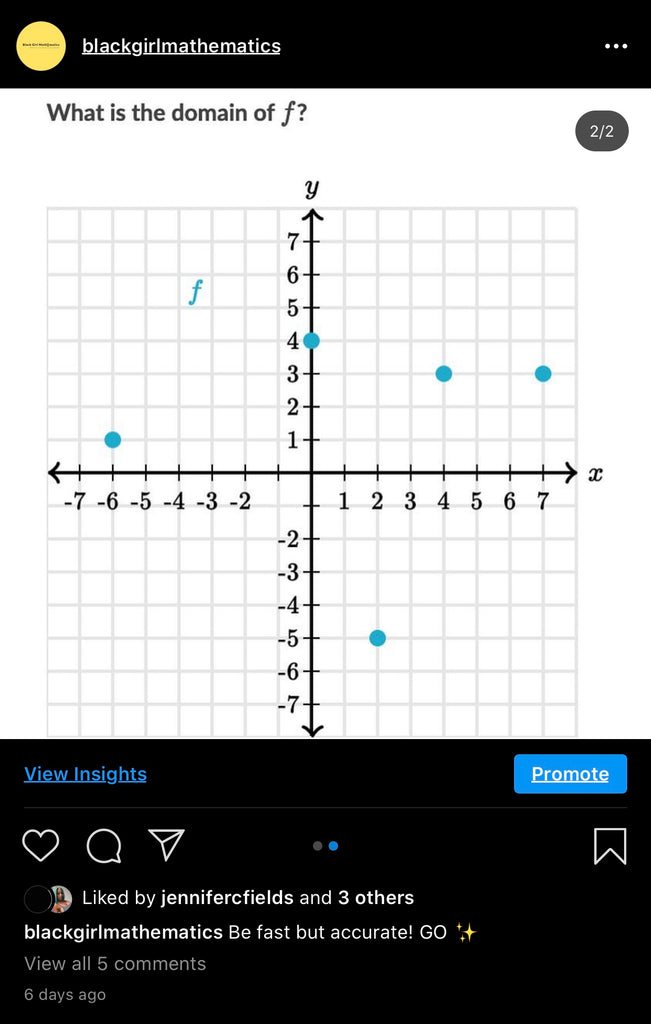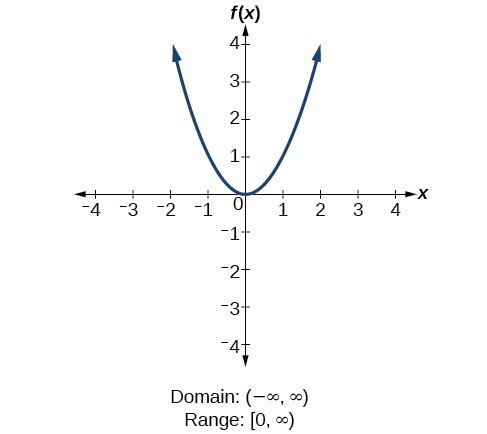# 8/5/2020 Winner08/05/2020 post: Finding domains of functions

Key words:
Domain
Function
Relation
Definitions:
Domain: is all the " x " values of any given function that can be plugged into the equation and get back a real number for y.
Example:
f(x) = x2Function: is a relation from a set of inputs to a set of possible outputs where each input is related to exactly one output.
Example:Relation: a set of ordered pairs.
Example: {(-1,6), (0,-6), (2,5)}

To answer the question above, "what is the domain of f" lets first identify if the function is in fact a function using the keywords above!
Relations of Graph A are {(-6,1), (0,4), (2,-5), (4,3), (7,3)} which is indeed a function due to inputs only giving out exactly one output. Given we know the relations we now know the domain, all x values {-6, 0, 4,  2, 4, 7}.

Tip: Another way to write domains is by using notations such as [ ] , ( ) , and ∞.
{-6, 0, 4,  2, 4, 7} can be written as [-6,7]
-
-
-
Winner: Josh from Palm Harbor, Florida won a virtual gift card to Amazon!
Congratulations Josh.